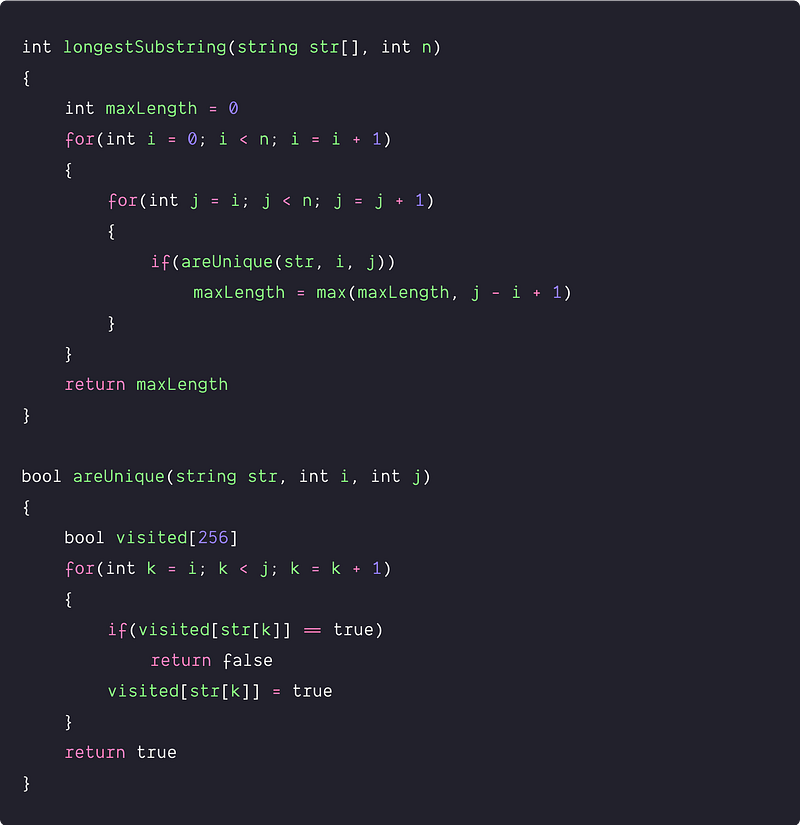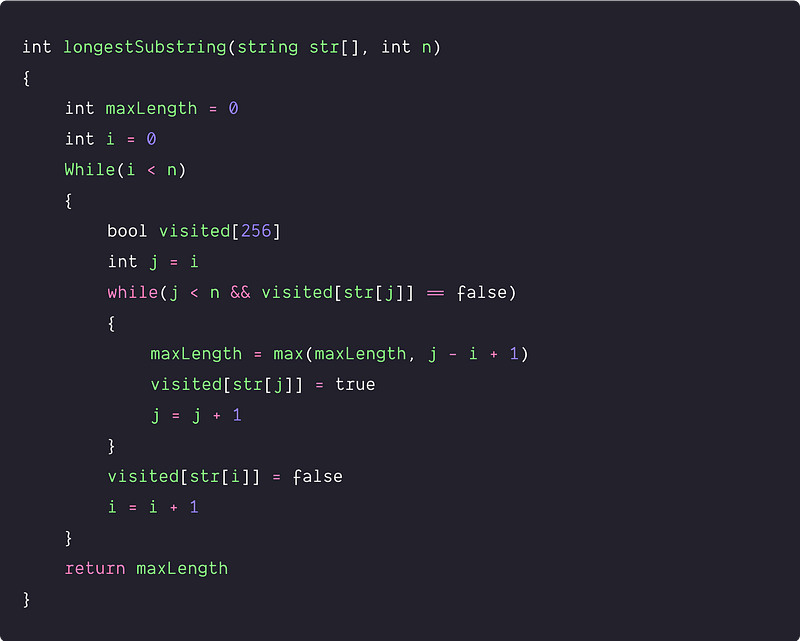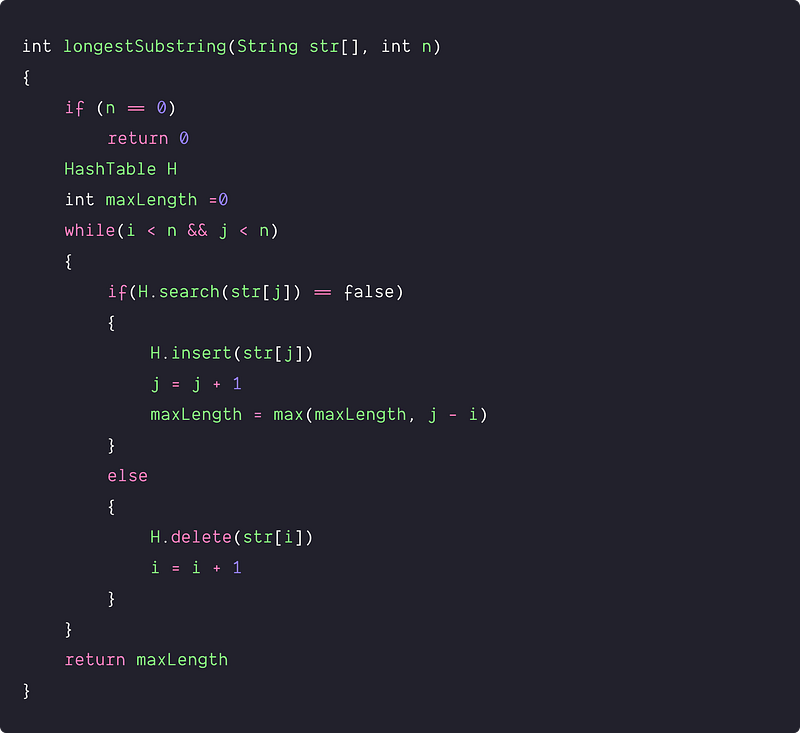# Longest Substring Without Repeating Characters

Difficulty: Medium, Asked-in: Microsoft, Amazon, Morgan Stanley

Key takeaway: An excellent problem to learn time complexity optimization using a sliding window approach. We can solve a lot of problems using a similar idea.

### Let’s understand the problem

Write a program to find the length of the longest substring without repeating characters.

• Substring is the continuous sub-part of the string, where we aim to determine the maximum subpart which has all the unique characters.
• Input string consists of English letters, digits, and symbols.
• The answer must be a continuous substring, not a subsequence of the string.
• There can be more than one longest substring (without repeating characters) of equal length. So, we need to return the length of the longest substring.

Example 1

Input: str = "abcbbcab", Output: 3

Explanation: There are three substrings of unique characters with the longest length 3: "abc", "bca", and "cab". So we return 3 as an output.

Example 2

Input: "bbbbb", Output: 1

Explanation: The answer is "b" with a length of 1.

Example 3

Input: "enjoyalgorithms", Output: 11

Explanation: "o" is the only repeating character, and the answer is "yalgorithms".

### Discussed solution approaches

• A brute force approach  using two nested loops
• Using sliding window approach
• An optimized sliding window approach

### A brute force approach  using two nested loops

Solution Idea and Steps

This is a simple approach where the idea is to explore all the substrings, and for each substring, we check whether it contains all unique characters or not. For a string with n characters, there will be n*(n+1)/2 substrings. (Think!)

1. We initialize a variable maxLength to keep track of the longest substring with unique characters. maxLength = 0
2. Now we explore all the possible substrings using two nested loops: outer loop from i = 0 to n-1 and inner loop from j = i to n-1. The idea is to pick starting character via the outer loop and explore all the substrings starting from that character using the inner loop.

• For each substring str[i, j] i.e. starting from index i and ending at index j, we use a function areUnique(str, i, j) to check if all the characters in the substring are unique or not. It will return true if all the characters are unique, otherwise false.
• If function areUnique(str, i , j) return true, we compare the maxLength with length of the substring. If(maxLength > j - i + 1), then we update the variable maxLength. Note: length of substring str[i, j] = j - i + 1.
3. By end of the nested loops, we return the value stored in maxLength.

Implementation of the function areUnique(str, i, j)

• To check if a substring has duplicate characters, we use a set visited to store the status of each possible character in the substring i.e. either true or false.
• We go through each character from index i to j using a loop. For each character, we check if it is already present in the set visited[] or not. If so, we return false. Otherwise, we update the status of that character true in the set visited[].
• By the end of the loop, we return true i.e. characters are unique in the substring.

Solution PseudocodeTime and space complexity analysis

• We are exploring all n(n+1)/2 substrings using nested loops.
• For each substring, function areUnique(str, i, j) would take O(j - i + 1) time to check the substring str[i, j] has unique characters or not.
• So overall time complexity = n(n+1)/2 * O( j - i +1) = O(n^2) * O( j - i +1). For an upper bound of the worst-case analysis, we consider O( j - i +1) as O(n). Why? Think! For a better understanding of such loop analysis, refer to this blog. So overall time complexity in the worst case = O(n^2) * O(n) = O(n^3)
• We are using few extra variables and a constant size set visited[]. So space complexity = O(1).

### Using  the sliding window technique

Solution Idea

Now a critical question is: how can we optimize the time complexity of the brute force approach? Think! The idea would be to apply the sliding window approach and track the repetition of characters in a substring i.e., whenever we found repetition in the current window, we slide the window and move to the next substring.

Here is an optimization insight: In the brute force idea, we repeatedly check a substring starting from the character str[i] to see if it has a duplicate character or not. We can optimize it further because, during the loop, if a substring str[i, j-1] is already checked to have no duplicate characters, then for the substring str[i, j], we only need to check if str[j] is already present in the substring str[i, j-1] or not.

We can use a hash set or extra array to check whether the character str[j] is there in the substring str[i, j-1] or not, which can be done in O(1) time complexity. But how do we implement this? Let's think! Note: A sliding window is an abstract concept commonly used in array/string problems. A window is a range of elements in the array/string usually defined by the start and ends indices, where the window “slides” in a forward direction by incrementing the left or right end.

Solution Steps

1. We initialise a variable maxLength to keep track of the longest substring with unique characters. maxLength = 0
2. We run an outer loop till i < n to explore the substring window starting from character str[i].
3. Now inside the loop, we initialize the right end of the window i.e. j = i. We also use an array visited as a hash set to store the status of characters in the current window [i, j). Initially i = j = 0.
4. Now we run an inner loop to check each substring in the window [i, j). This loop will run till j < n && visited[str[j]] == false.

• If str[j] is not present in the window [i, j) i.e. if(visited[str[j]] == false): we mark the str[j] in the visited[] and keep track of the longest substring length. Now we slide the current window to one right by incrementing j and continue the same process in the inner loop till str[j] is not present in the window [i, j).
• If the character str[j] is already present or visited in the current window, we increment i by 1 and go to the next iteration of the outer loop. Now we check the new window of a substring starting from index i + 1. Note: before moving to the next iteration of the outer loop, we should set the status of the character str[i] in the set visited[] i.e. visited[str[i]] = false. (Think!)
5. The outer loop will stop when the left end of the sliding window reaches the end of the string i.e., i = n. So, we return the value stored in the variable maxLength.

Solution PseudocodeTime and space complexity analysis

We are using two nested loops and performing an O(1) operation at each iteration. The worst-case occur when the inner loop runs till the end every time. Or in other words, the worst-case will occur when all the characters in the string are unique. Think! In such a situation, the nested loop will explore all possible substrings i.e. n(n+1)/2. Time complexity = n(n+1)/2 * O(1) = O(n^2).

We are using a constant size set visited[] of size 256, so space complexity = O(1).

### An optimized sliding window solution

Solution Idea

Now the critical question is: can we optimize the above approach further and solve this problem in O(n) time complexity? Can we think of removing the inner loop of the previous approach? Here is a hint:

• At each iteration of the outer loop in the previous approach, we ignore the previous window [i, j) and reset j = i to start a new window. In other words, we are starting with a new window of size 1 at each iteration of the outer loop.
• If characters in the window [i, j) are unique, then characters in the window [i+1, j) will also be unique. So in the next iteration of the outer loop, there is no need to start with a new window of size 1 or reset j = i (Think!).

Optimzed Solution Steps

• Scan the string from left to right using two window pointers i and j. Also, keep track of the longest length of the substring using the variable maxLength and maintain a hash table to keep track of visited characters.
• If character str[j] is not present in the hash table, we insert it into the hash table and slide the current window right by incrementing the pointer j. We also update the longest length of the substring with unique characters i.e. maxLength = max (maxLength, j - i).
• If character str[j] is already present in the hash table, we delete the character str[j] from the hash table and slide the window right by incrementing pointer i.
• This process will end when any one of the pointers reaches the end of the string i.e. i = n or j = n. At this stage, we return the value stored in the variable maxLength.

Solution PseudocodeTime and space complexity analysis

At each iteration of the while loop, we either increment pointer i or pointer j, based on the comparison if(H.search(str[j]) == false). So the loop will run n times and perform a constant number of operations at each iteration. Time complexity = O(n). The algorithm requires O(n) space for the hash table, so space complexity = O(n)

### Critical ideas to think!

• Can we optimize the efficient approach further and solve it using O(1) extra space?? Instead of a hash table, can we use a set visited to store the status of each character in a substring window?
• How do we modify the above approaches to print the longest substring in lexicographic order?
• Try to explore the precise analysis of the brute force approach?
• Explore patterns of problem-solving using the sliding window.

### Comparison of time and space complexities

• Using two nested loops: Time = O(n^3), Space = O(1)
• Using sliding window approach: Time = O(n^2), Space = O(1)
• An optimized sliding window approach: Time = O(n), Space = O(n)

### Suggested coding problems to practice

Enjoy learning, Enjoy algorithms!

Get well-designed application and interview centirc content on ds-algorithms, machine learning, system design and oops. Content will be delivered weekly.